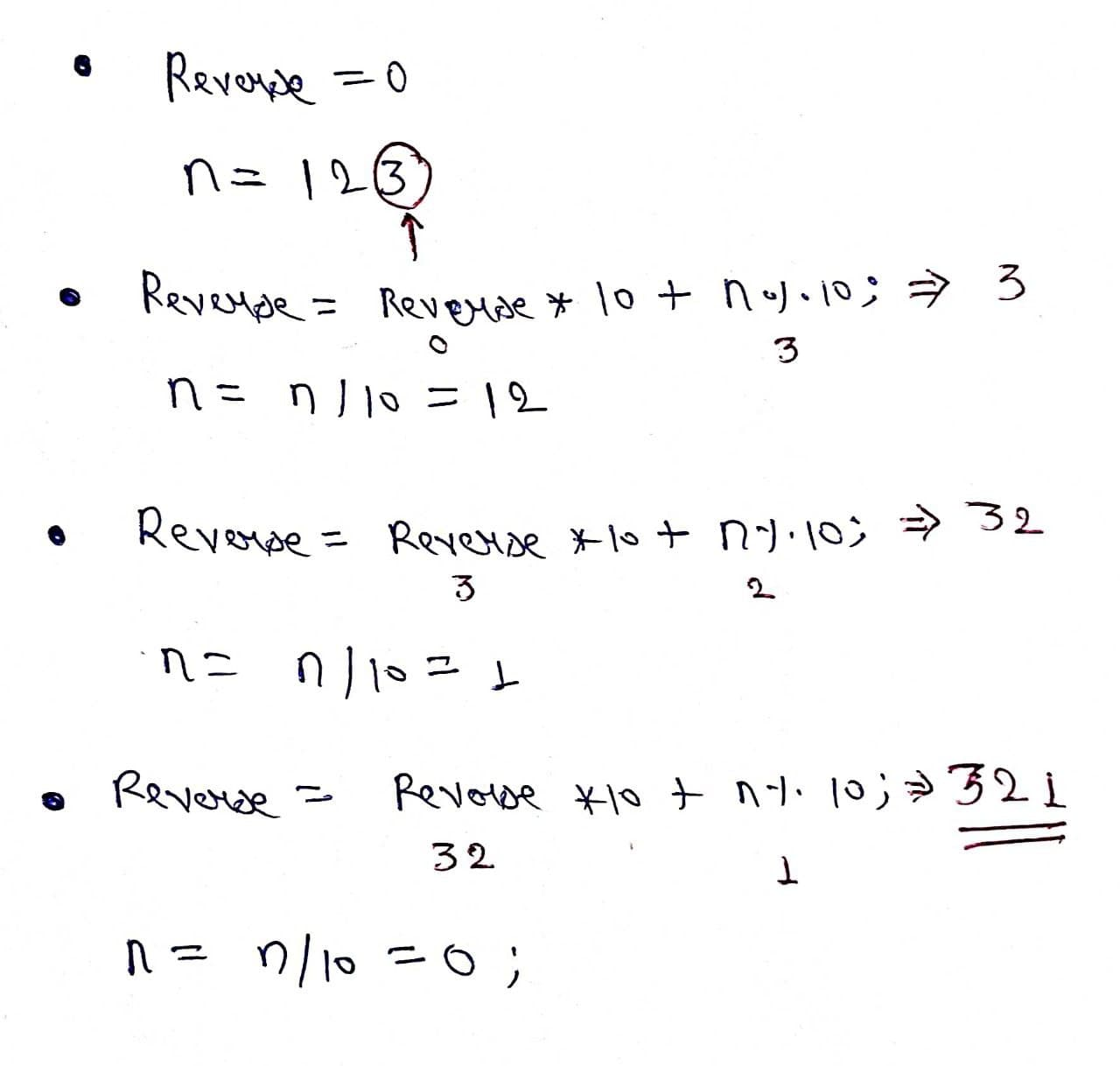# Find all Palindrome Numbers in a given range

Problem Statement: Given a range of numbers, find all the palindrome numbers in the range.

Note: A palindromic number is a number that remains the same when its digits are reversed.OR  a palindrome is a number that reads the same forward and backward Eg: 121,1221, 2552

Examples:

```Example 1:
Input: min = 10 , max = 50
Output: 11 22 33 44
Explanation: 11, 22, 33, 44 will remain the same when they read from forward or backward.

Example2:
Input: min = 100 , max = 150
Output: 101 111 121 131 141
Explanation: 11, 22, 33, 44 will remain the same when they read from forward or backward.
```

### Solution

Disclaimer: Don’t jump directly to the solution, try it out yourself first.

Intuition: We run a for loop, and check for every number individually, if it is palindrome then we will print it.

Palindrome: We will reverse the number, if it is equal to the given number then it is palindrome otherwise it is not.

Approach:

• Use a for loop and pass every element to the palindrome function.
• Now make a palindrome function which takes an integer as a parameter.
• Reverse the given number.
• If the reversed number is equal to the given number then print it.

Reversing a number:

• Make a result variable.
• Take the last digit of the element.
• Now multiple variable by 10 and add the last digit.
• Repeat till element>0.Code:

## C++ Code

``````#include<iostream>
using namespace std;

// A function to check if n is palindrome
bool isPalindrome(int n)
{
int reverse = 0;
int temp = n;
while(temp>0) {
reverse = reverse*10 + temp%10;
temp = temp/10;
}

// If n and reverse are same,
// then n is palindrome
if(n==reverse)return true;
return false;
}

int main()
{
int min = 100;
int max = 150;
for(int i=min; i<=max; i++) {
if(isPalindrome(i)) {
cout<<i <<" ";
}
}
return 0;
}
``````

Output: 101 111 121 131 141

Time Complexity: O(N)

Space Complexity: O(1)

## Java Code

``````import java.util.*;

public class tuf {
static boolean isPalindrome(int n)
{

int reverse = 0;
int temp = n;
while(temp>0) {
reverse = reverse*10 + temp%10;
temp = temp/10;
}

// If n and reverse are same,
// then n is palindrome
if(n==reverse)return true;
return false;
}

public static void main(String args[])
{
int min = 100;
int max = 150;
for(int i=min; i<=max; i++) {
if(isPalindrome(i)) {
System.out.print(i + " ");
}
}

}
}
``````

Output: 101 111 121 131 141

Time Complexity: O(N)

Space Complexity: O(1)

Special thanks to Prashant Sahu for contributing to this article on takeUforward. If you also wish to share your knowledge with the takeUforward fam, please check out this article